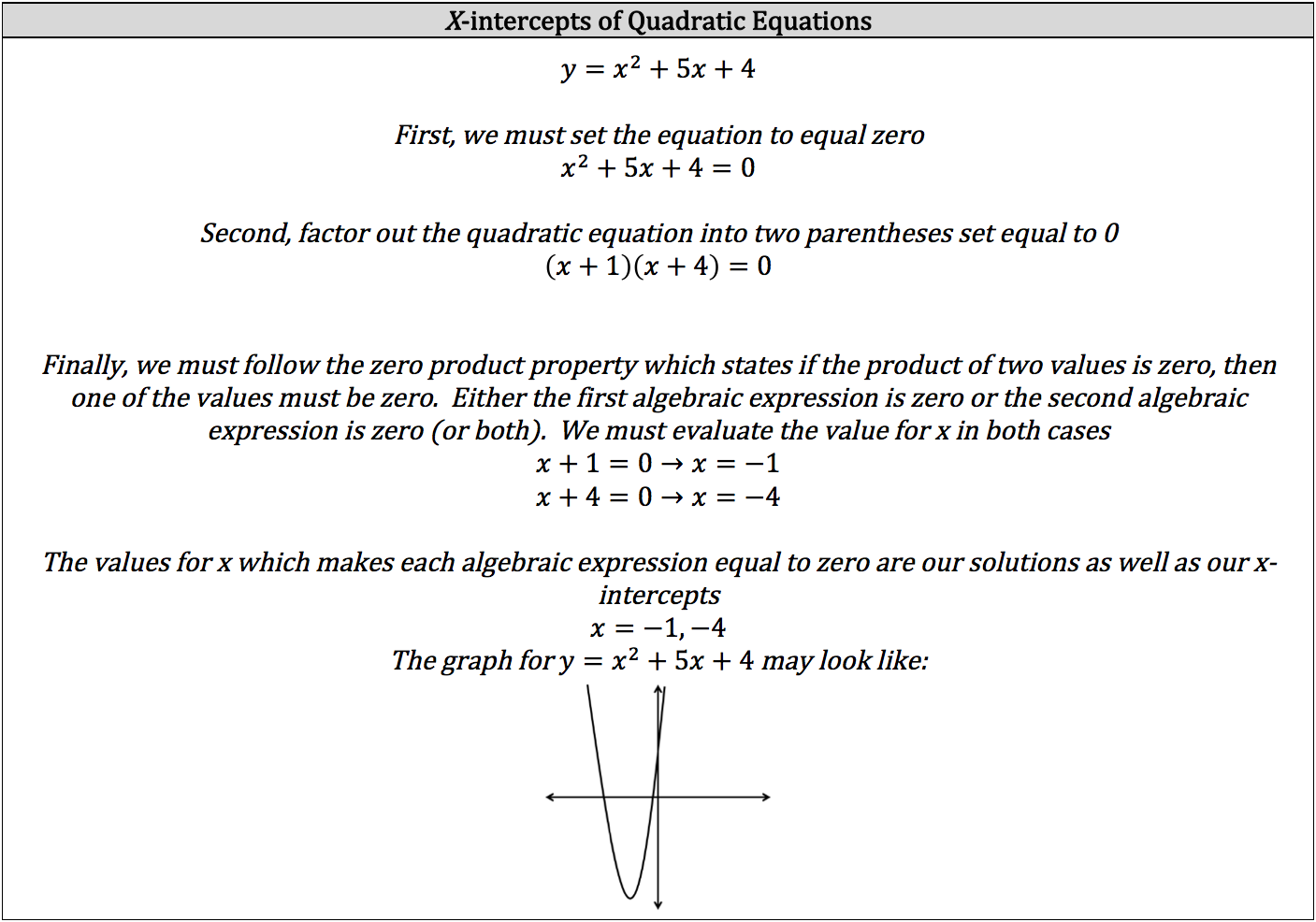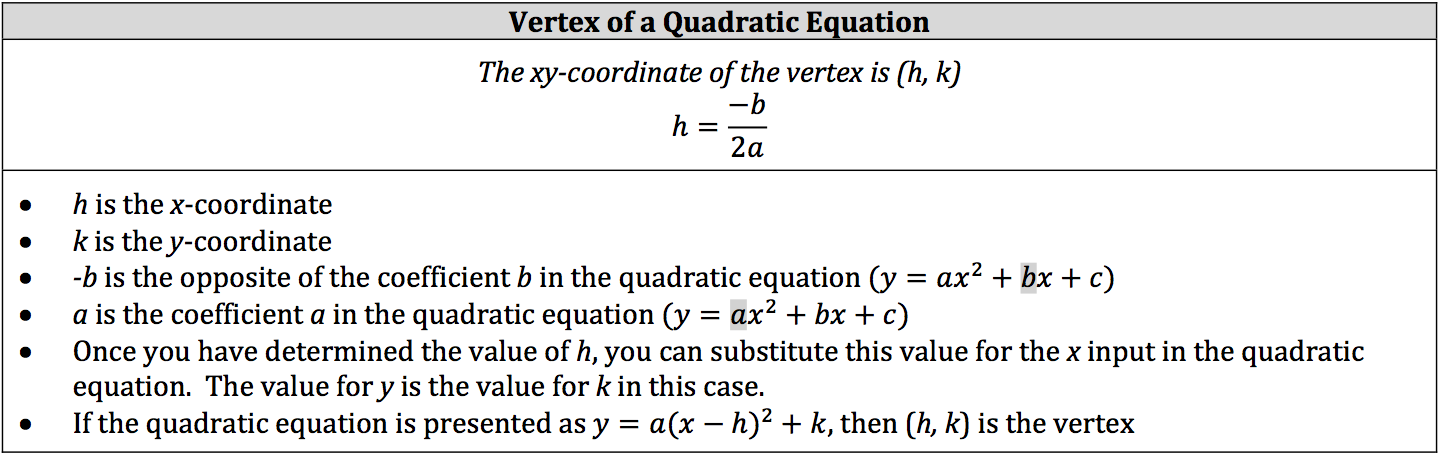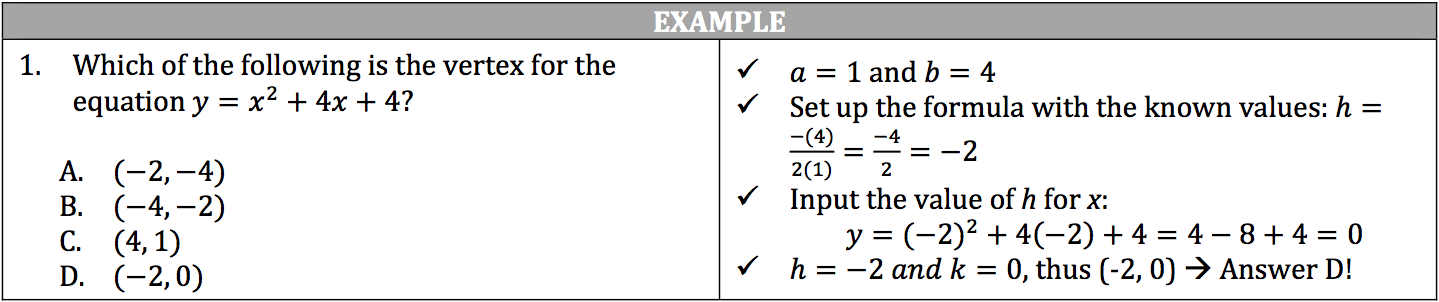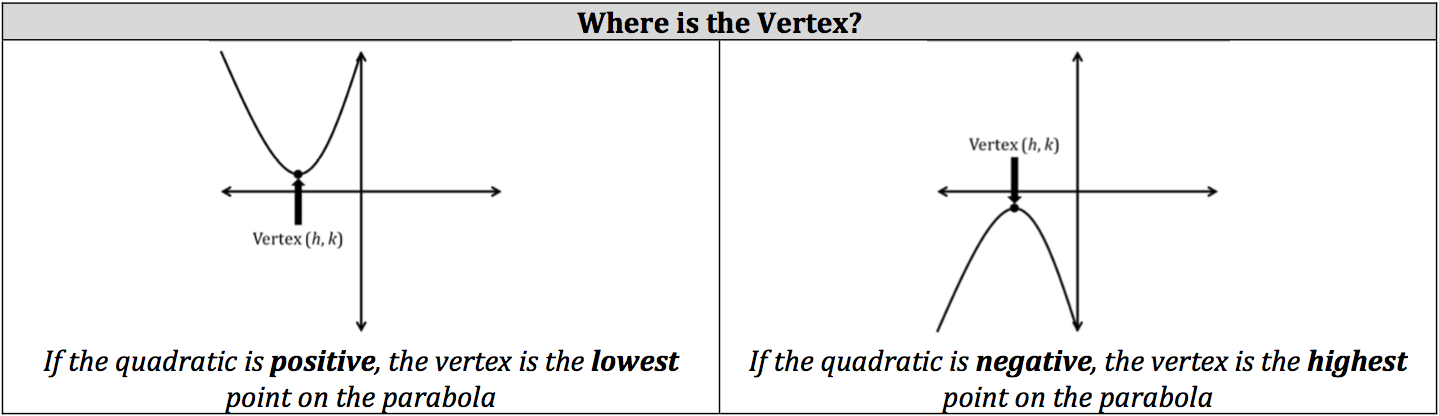Quadratic equations function in the same manner as linear equations in that you have a particular input resulting in a particular output. You plot points on the graph in the same manner as you do linear equations. The difference is that the graph of a quadratic forms a curved line, called a parabola, instead of a straight line. You will not need to know the quadratic formula for the ISEE.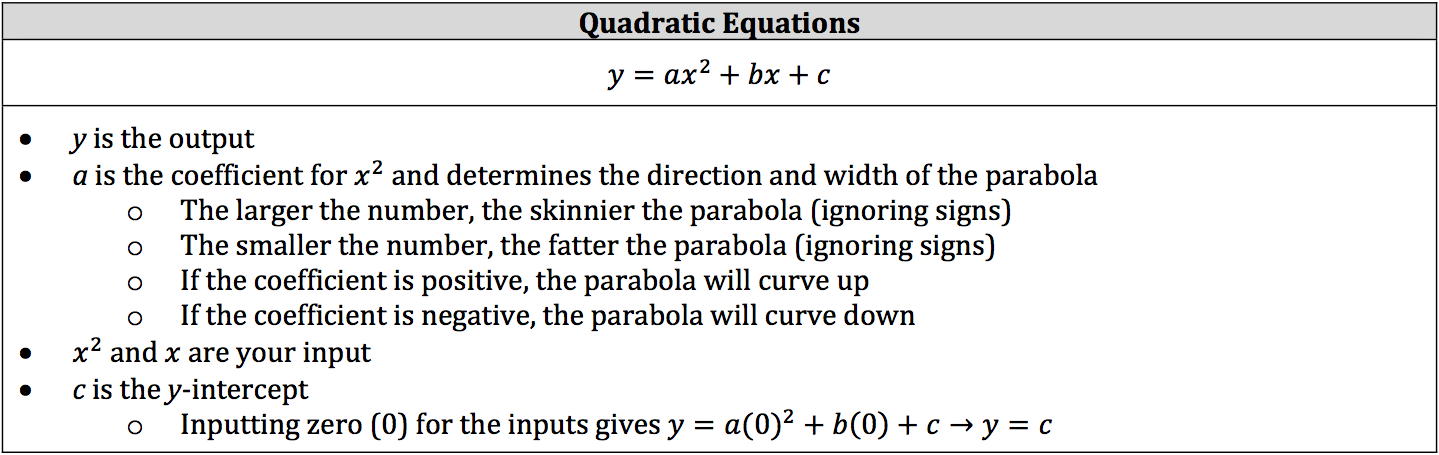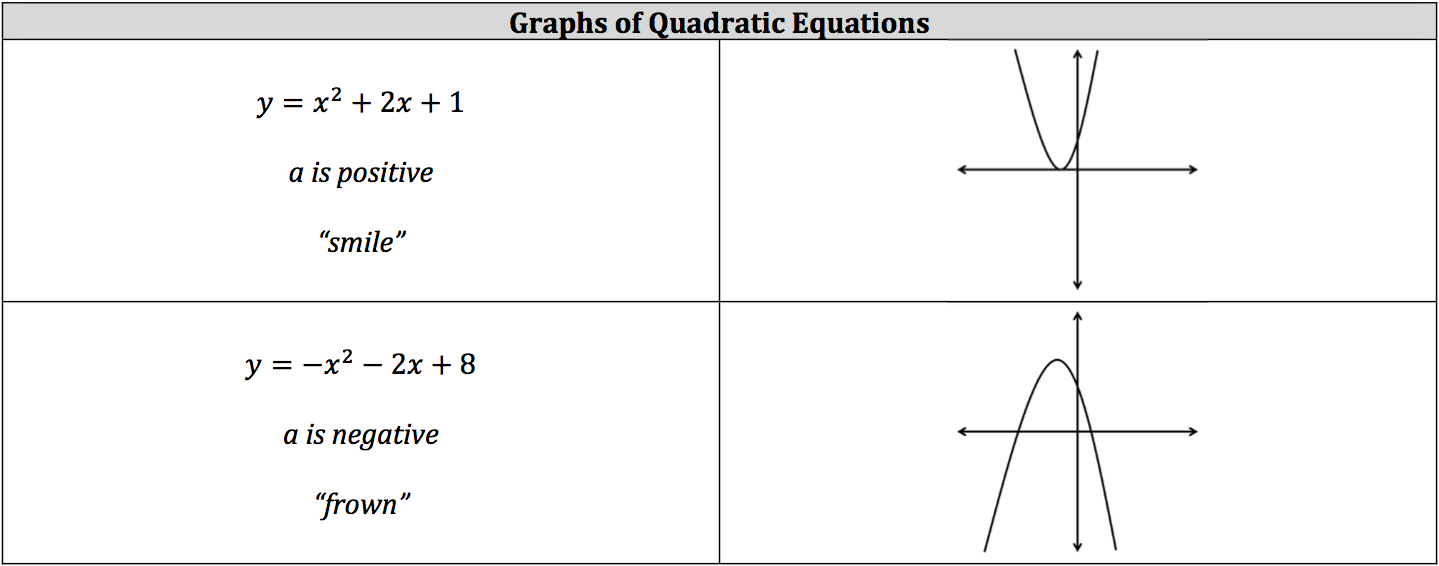Aside from knowing what a quadratic equation will look like on a graph, you will need to know how to find its x‐intercepts and its vertex (the highest or lowest point of a parabola). To find a quadratic equation’s x‐ intercepts, the quadratic equation must be set equal to zero (0). The reason for this is that for any coordinate that falls on the x‐axis, the value of y will be zero (ex. (3, 0) or (‐3, 0)). This is also true for solving for x for quadratic equations, since it is the same thing. If your quadratic equation is set to anything other than zero, you must manipulate the quadratic equation to equal zero.